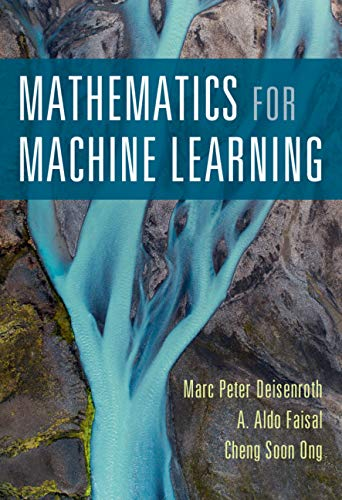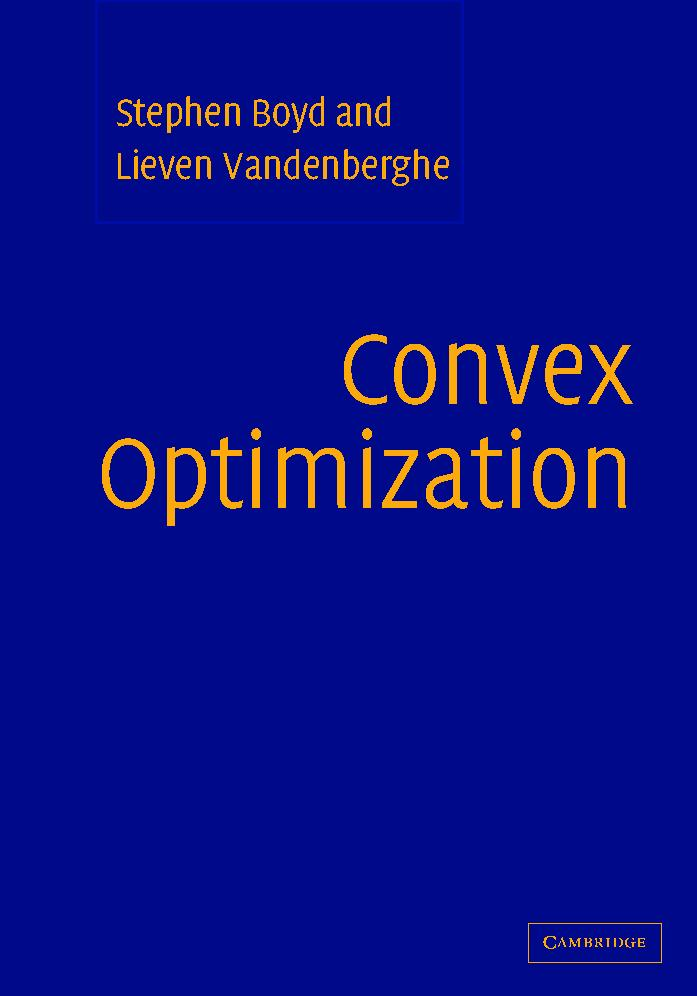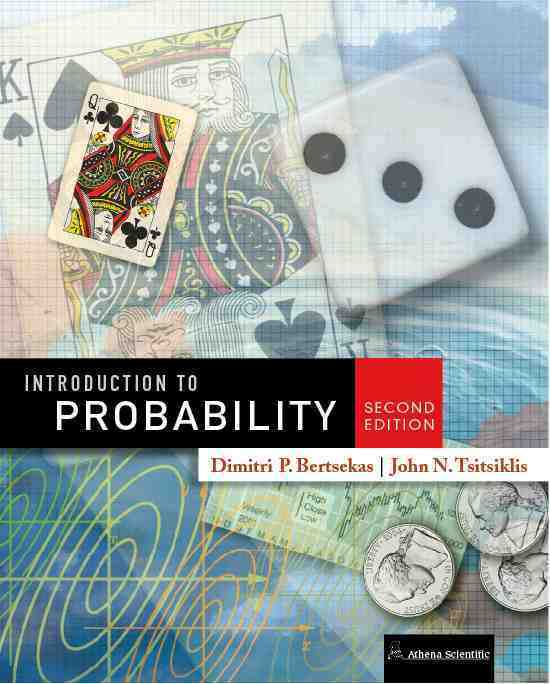Back to Yung Yi, Courses

Textbook

- Introduction to Probability, 2nd edition by Dimitri P. Bertsekas and John N. TsitsiklisThey have been made by Prof. Yung Yi, and they keep being updated by him. He has used most of the contents from the textbook, but more explanatinos/figures/examples have been added if necessary. In using the figures of the main textbook, he did not leave any courtesy in the slides, but for other figures, he has tried to mention their sources. The lecture notes are being made in the overleaf platform. How to compile them and generate the various formats of prints is described in the introduction.

Contents   Material

1. Introduction and Overview

• Contents
• Suggestions for course schedules
• Target audience
• Organization of LaTex source files (e.g., how to compile etc)
• Lecture slides
• For prints: 1, 2, 4
• 2. Linear Algebra

• Systems of Linear Equations
• Matrices
• Solving Systems of Linear Equations
• Vector Spaces
• Linear Independence
• Basis and Rank
• Linear Mappings
• Affine Spaces
• Lecture slides
• For prints: 1, 2, 4
• 3. Analytic Geometry

• Norms
• Inner Products
• Lengths and Distances
• Angles and Orthogonality
• Orthonormal Basis
• Orthogonal Complement
• Inner Product of Functions
• Orthogonal Projections
• Rotations
• Lecture slides
• For prints: 1, 2, 4
• 4. Matrix Decomposition

• Determinant and Trace
• Eigenvalues and Eigenvectors
• Cholesky Decomposition
• Eigendecomposition and Diagonalization
• Singular Value Decomposition
• Matrix Approximation
• Matrix Phylogeny
• Lecture slides
• For prints: 1, 2, 4
• 5. Vector Calculus

• Differentiation of Univariate Functions
• Useful Identities for Computing Gradients
• Backpropagation and Automatic Differentiation
• Higher-Order Derivatives
• Linearization and Multivariate Taylor Series
• Lecture slides
• For prints: 1, 2, 4
• 6. Probability and Distributions

• Construction of a Probability Space
• Discrete and Continuous Probabilities
• Sum Rule, Product Rule, and Bayes’ Theorem
• Summary Statistics and Independence
• Gaussian Distribution
• Conjugacy and the Exponential Family
• Change of Variables/Inverse Transform
• Lecture slides
• For prints: 1, 2, 4
• 7. Optimization

• Constrained Optimization and Lagrange Multipliers
• Convex Sets and Functions
• Convex Optimization
• Convex Conjugate
• Lecture slides
• For prints: 1, 2, 4
• 8. When Models Meet Data

• Data, Models, and Learning
• Models as Functions: Empirical Risk Minimization
• Models as Probabilistic Models: Parameter Estimation (ML and MAP)
• Probabilistic Modeling and Inference
• Directed Graphical Models
• Model Selection
• Lecture slides
• For prints: 1, 2, 4
• 9. Linear Regression

• Problem Formulation
• Parameter Estimation: ML
• Parameter Estimation: MAP
• Bayesian Linear Regression
• Maximum Likelihood as Orthogonal Projection
• Lecture slides
• For prints: 1, 2, 4
• 10. Dimensionality Reduction with Principal Component Analysis

• Problem Setting
• Maximum Variance Perspective
• Projection Perspective
• Eigenvector Computation and Low-Rank Approximations
• PCA in High Dimensions
• Key Steps of PCA in Practice
• Latent Variable Perspective
• Lecture slides
• For prints: 1, 2, 4
• 11. Density Estimation with Gaussian Mixture Models

• Gaussian Mixture Model
• Parameter Learning: MLE
• Latent-Variable Perspective for Probabilistic Modeling
• EM Algorithm
• Lecture slides
• For prints: 1, 2, 4
• 12. Classification with Support Vector Machines

• Story and Separating Hyperplanes
• Primal SVM: Hard SVM
• Primal SVM: Soft SVM
• Dual SVM
• Kernels
• Numerical Solution
• Lecture slides
• For prints: 1, 2, 4